## Solving Simple Equations

When solving a simple equation, think of the equation as a balance, with the equals sign (=) being the fulcrum or center. Thus, if you do something to one side of the equation, you must do the same thing to the other side. Doing the same thing to both sides of the equation (say, adding 3 to each side) keeps the equation balanced.

Solving an equation is the process of getting what you're looking for, or solving for, on one side of the equals sign and everything else on the other side. You're really sorting information. If you're solving for x, you must getx on one side by itself.

Some equations involve only addition and/or subtraction.

##### Example 1

Solve for x.

x + 8 = 12

To solve the equation x + 8 = 12, you must get x by itself on one side. Therefore, subtract 8 from both sides.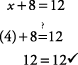##### Example 2

Solve for y.

y – 9 = 25

To solve this equation, you must get y by itself on one side. Therefore, add 9 to both sides.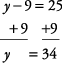To check, simply replace y with 34:##### Example 3

Solve for x.

x + 15 = 6

To solve, subtract 15 from both sides.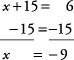To check, simply replace x with –9 :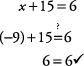Notice that in each case above, opposite operations are used; that is, if the equation has addition, you subtract from each side.

#### Multiplication and division equations

Some equations involve only multiplication or division. This is typically when the variable is already on one side of the equation, but there is either more than one of the variable, such as 2 x, or a fraction of the variable, such as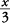orIn the same manner as when you add or subtract, you can multiply or divide both sides of an equation by the same number, as long as it is not zero, and the equation will not change.

##### Example 4

Solve for x.

x = 9

Divide each side of the equation by 3.To check, replace x with 3: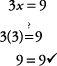##### Example 5

Solve for y.To solve, multiply each side by 5.To check, replace y with 35:##### Example 6

Solve for x.To solve, multiply each side by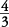.Or, without canceling,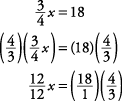Notice that on the left you would normally not write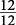because it would always cancel to 1 x, or x.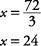#### Combinations of operations

Sometimes you have to use more than one step to solve the equation. In most cases, do the addition or subtraction step first. Then, after you've sorted the variables to one side and the numbers to the other, multiply or divide to get only one of the variables (that is, a variable with no number, or 1, in front of it: x, not 2 x).

##### Example 7

Solve for x.

x + 4 = 10

Subtract 4 from both sides to get 2 x by itself on one side.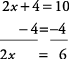Then divide both sides by 2 to get x.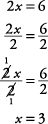##### Example 8

Solve for x.

5x – 11 = 29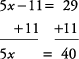Divide each side by 5.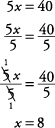To check, replace x with 8: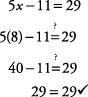##### Example 9

Solve for x.Subtract 6 from each side.Multiply each side by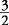.To check, replace x with 9: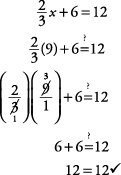##### Example 10

Solve for y.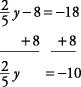Multiply each side by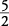.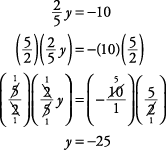To check, replace y with –25: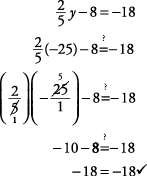##### Example 11

Solve for x.

x + 2 = x + 4

Subtract 2 from both sides (which is the same as adding –2).Subtract x from both sides.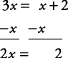Note that 3 x – x is the same as 3 x – 1 x.

Divide both sides by 2.To check, replace x with 1:##### Example 12

Solve for y.

y + 3 = 2 y + 9

Subtract 3 from both sides.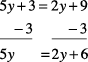Subtract 2 y from both sides.Divide both sides by 3.To check, replace y with 2: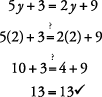Sometimes you need to simplify each side (combine like terms) before actually starting the sorting process.

##### Example 13

Solve for x.

x + 4 + 2 = 12 + 3

First, simplify each side.Subtract 6 from both sides.Divide both sides by 3.To check, replace x with 3: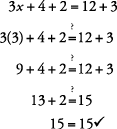##### Example 14

Solve for x.

x + 2 x + 4 = 5 x + 3 + 11

Simplify each side.

x + 4 = 5 x + 14

Subtract 4 from both sides.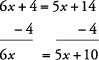Subtract 5 x from both sides.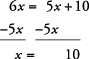To check, replace x with 10: